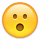# help me with a math problem

Member, Mobile Tester Posts: 915
This will probably turn out to have an easy answer, but math isn't my strong suit:
Say the d20 is missing from my set of dice, but I want to replicate the range of 1-20. Easy enough...roll a d4, d8, and d10 together to get a range of 3-22, then subtract 2 to get the 1-20 range. My question is, is that actually equivalent to rolling a d20, or will the chance of each number coming up be weighted towards the numbers in the middle, and how do you figure that out?
Thanks!

• No, it's not equivalent to rolling a d20. In a d20 there is an even distribution of values. In your case, you can roll a 10 in many different ways, for example. Hence, it will be weighed towards the middle.
• Member, Mobile Tester Posts: 915
That's what I thought...how does one show that mathematically?
• What do you need to show? That the distribution is different? Or what the distributions are? It's easy to show that rolling a 1 in your scenario is way unlikelier than in d20. Rolling a 1 means rolling a 1 in each separate roll.

P(1) = 1/4 * 1/8 * 1/10 = 1/320

Whereas in d20

P(1) = 1/20
• And Microsoft Excel has a random number generator built in. If you put
=RANDBETWEEN(1,20)
into a cell and click delete in an empty cell, it will give you a random number between 1 and 20.
• procco said:

This will probably turn out to have an easy answer, but math isn't my strong suit:
Say the d20 is missing from my set of dice, but I want to replicate the range of 1-20. Easy enough...roll a d4, d8, and d10 together to get a range of 3-22, then subtract 2 to get the 1-20 range. My question is, is that actually equivalent to rolling a d20, or will the chance of each number coming up be weighted towards the numbers in the middle, and how do you figure that out?
Thanks!

No, it's not the same. A d20 has exactly the same probability of getting each number: 5%. Using your suggested method, the probability of getting a 1 or 20 is 1/(4x8x10), which works out at about 0.3%, while the probability of getting 10 or 11 is much higher (to early in the day for me to work out exactly what).
• edited January 2017

And Microsoft Excel has a random number generator built in. If you put
=RANDBETWEEN(1,20)
into a cell and click delete in an empty cell, it will give you a random number between 1 and 20.

There's a button on the Formula tab called "Calculate Sheet" that will do the same as a delete. If you're going to do any extensive die rolling in Excel, I would recommend setting calculation options to "Manual". That way you won't accidently reroll the dice and lose a roll you wanted to keep. I use Excel for all my character creation die rolls. I like it because you can set up complicated rolls like "roll 4d6 and drop 1".

Edit: There's a lot of free dice rolling apps for Android and, I assume, iOS. I played around with this one for a few minutes and it seems OK.Post edited by BillyYank on
• Member, Mobile Tester Posts: 915
Thanks, fellas. I didn't actually lose my d20, it was just something I was curious about for no good reason. I understand that the numbers towards the middle of the scale are going to have a higher chance of coming up since there are more ways of them to come up...I'm going to try to work out on my own how to figure out what the chance of each number is, then check back to see if anyone has a solution for that...
• Member, Translator (NDA) Posts: 515
Then throwing three d6s at once vs. d6 three times in a row is different?Anyway i think cannot replace d20 with two d10s, alas I cannot prove mathematically but just intuition.
• Teflon said:

Then throwing three d6s at once vs. d6 three times in a row is different?Anyway i think cannot replace d20 with two d10s, alas I cannot prove mathematically but just intuition.

You can, but don't add them. Use them as percentile dice (one d10 for the tens place, one d10 for the ones place).

01-05 = 1
06-10 = 2
11-15 = 3
etc..
96-00 = 20
• @procco It's simple. It's the number of combinations that result in a roll over the total number of combinations:

P(1) = 1 / 320, because there is only one way to get 3 on 1d4+1d8+1d10
P(2) = 3 / 320, because there are 3 ways to get 4 on 1d4+1d8+1d10

and so on. Of course, finding out the number of combinations may not be so simple• Member, Translator (NDA) Posts: 515: What school of magic are you?
AstroBryGuy : Math.
• The 1d4 + 1d8 + 1d10 - 2 method comes out as a standard bell curve:

http://anydice.com/program/a8fc